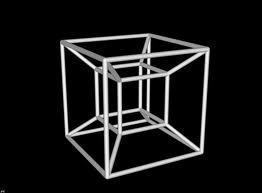•• # What Are the Four Dimensions?

The four dimensions are concepts in mathematics to describe different shapes. It is easy to understand a first dimension, second dimension and third dimension shape. All things are 3D in the world, but images can be 2D. A first dimension is as easy as a line. However, what is the fourth dimension? The fourth dimension is an abstract concept that takes two third dimension shapes and connects them together.

## What is the First Dimension?

The first dimension is an interval. It is the line between two points. So, drawing a line on a map from New York City to Boston would have the interval being the dimension and then the two points–New York City and Boston–would be the two faces of that interval. The distance is irrelevant; all that matters is that it is some distance–perhaps called L for its value–that is between two specific points.

## What is the Second Dimension?

The second dimension is what would be referred to as a 2D image. The second dimension takes the first interval and then drags it over in a parallel fashion. So, when making a cube, for example, the first interval would be dragged over an equal distance to the right or left or top and bottom. Once that is done, the two intervals are connected at their points creating a square (or whatever shape you were going for.)To get the number of faces, simply multiply the number of dimensions by the number of faces per interval. So, two faces per interval times two dimensions means that there are four faces to a second dimension cube.

## What is the Third Dimension?

The third dimension is the cube most people are used to. To make this, the second dimension square is dragged in a distance above or below the initial second dimension image. Then, lines are drawn to connect these two second dimension squares together. When this is done, a cube is formed. This is what a 3D image looks like because it has more than just lengths and heights. It now has a depth.

To get the number of faces, multiply the number of dimensions by the number of faces. Each dimension is always going to have two faces because that goes back to the initial one where an interval was surrounded by a face or a point on each side. Therefore, a third dimension shape has six faces.

## What is the Fourth Dimension?

The fourth dimension is called a tesseract. To achieve this, we take a third dimension image and then drag it an L distance into the four dimension. Then, connect the lines to create a fourth dimension image. Because it is the fourth dimension and each dimension has two faces, the total number of faces for this is 8 faces. Drawing is very simple. Draw two cubes on a piece of paper and then connect the corresponding corners. This creates a fourth dimension image.

1. #### Arnold

18 June, 2018 at 8:38 pm

We live in our three dimensional world with the phenomenon of observation–our fourth dimension…

That observation shows us expansion points in opposite directions–towards ourselves towards the universe…

Without observation could humanity or dimensions exist…Is Being here now a cosmological constant…